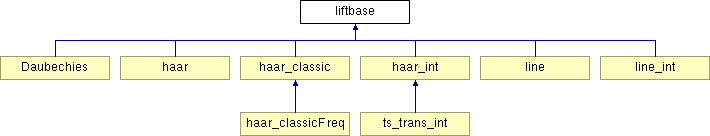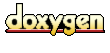Main Page   Class Hierarchy   Compound List   File List   Compound Members   File Members

# liftbase Class Template Reference

This is the base class for simple Lifting Scheme wavelets using split, predict, update or update, predict, merge steps. More...

`#include <liftbase.h>`

Inheritance diagram for liftbase::List of all members.

## Public Methods

virtual void forwardStep (T &vec, const int n)
One step in the forward wavelet transform. More...

virtual void forwardStepRev (T &vec, const int N)
Reverse forward transform step. More...

virtual void forwardTrans (T &vec, const int N)
Simple wavelet Lifting Scheme forward transform. More...

virtual void inverseStep (T &vec, const int n)
One inverse wavelet transform step. More...

virtual void inverseStepRev (T &vec, const int n)
Reverse inverse transform step. More...

virtual void inverseTrans (T &vec, const int N)
Default two step Lifting Scheme inverse wavelet transform. More...

## Protected Types

enum  transDirection { forward = 1, inverse = 2 }

## Protected Methods

void split (T &vec, int N)
Split the vec into even and odd elements, where the even elements are in the first half of the vector and the odd elements are in the second half. More...

void merge (T &vec, int N)
Merge the odd elements from the second half of the N element region in the array with the even elements in the first half of the N element region. More...

virtual void predict (T &vec, int N, transDirection direction)=0
Predict step, to be defined by the subclass. More...

virtual void predictRev (T &vec, int N, transDirection direction)
Reverse predict step. More...

virtual void update (T &vec, int N, transDirection direction)=0
Update step, to be defined by the subclass. More...

virtual void updateRev (T &vec, int N, transDirection direction)
Reverse update step. More...

## Detailed Description

### template<class T, class T_elem> class liftbase

This is the base class for simple Lifting Scheme wavelets using split, predict, update or update, predict, merge steps.

Simple lifting scheme wavelets consist of three steps, a split/merge step, predict step and an update step:

• The split step divides the elements in an array so that the even elements are in the first half and the odd elements are in the second half.

• The merge step is the inverse of the split step. It takes two regions of an array, an odd region and an even region and merges them into a new region where an even element alternates with an odd element.

• The predict step calculates the difference between an odd element and its predicted value based on the even elements. The difference between the predicted value and the actual value replaces the odd element.

• The predict step operates on the odd elements. The update step operates on the even element, replacing them with a difference between the predict value and the actual odd element. The update step replaces each even element with an average. The result of the update step becomes the input to the next recursive step in the wavelet calculation.

The split and merge methods are shared by all Lifting Scheme wavelet algorithms. This base class provides the transform and inverse transform methods (forwardTrans and inverseTrans). The predict and update methods are abstract and are defined for a particular Lifting Scheme wavelet sub-class.

This is a template version of the lifting scheme base class. The template must be instantiated with an array or an object that acts like an array. Objects that act like arrays define the left hand side and right hand side index operators: []. To allow wavelet transforms based on this base class to be used with the wavelet packet transform, this class makes public both the forward and inverse transforms (forwardTrans and inverseTrans) and the forward and inverse transform steps (forwardStep and inverseStep). These "step" functions are used to calculate the wavelet packet transform.

Instantiating the Template

The liftbase template takes two type arguments:

1. The type of the array or '[]' operator indexable object.
2. The type of the data element.

The simplest example is a wavelet class derived from an instance of the liftbase tempate which takes a double array and has a double element type. This declaration is shown below:

```  class Haar : public liftbase<double *, double>
```

An object type can be used for the first template argument, as long as the object supports the '[]' operator, which returns an element whose type is defined by the second argument. In the example below, the packcontainer '[]' operator returns a double.

```  class Poly : public liftbase<packcontainer, double>
```

References:

Author:
Ian Kaplan

Definition at line 155 of file liftbase.h.

## Member Enumeration Documentation

 template enum liftbase::transDirection` [protected]`

Enumeration values:
 forward "enumeration" for forward wavelet transform. inverse "enumeration" for inverse wavelet transform.

Definition at line 159 of file liftbase.h.

```00159                {
00161     forward = 1,
00163     inverse = 2
00164   } transDirection;
```

## Member Function Documentation

 template void liftbase::forwardStep ( T & vec, const int n )` [inline, virtual]`
 One step in the forward wavelet transform. Reimplemented in Daubechies, and haar. Definition at line 262 of file liftbase.h. Referenced by forwardTrans(), Daubechies::forwardTrans(), packtree_base_int::newLevel(), and packtree_base::newLevel(). ```00263 { 00264 split( vec, n ); 00265 predict( vec, n, forward ); 00266 update( vec, n, forward ); 00267 } // forwardStep ```

 template void liftbase::forwardStepRev ( T & vec, const int n )` [inline, virtual]`
 Reverse forward transform step. The result of the high pass filter is stored in the lower half of the array and the result of the low pass filter is stored in the upper half. This function should be defined by any subclass that is used for wavelet frequency analysis. Reimplemented in Daubechies, and haar_classicFreq. Definition at line 278 of file liftbase.h. Referenced by packtree_base_int::newLevel(), and packtree_base::newLevel(). ```00279 { 00280 assert(false); 00281 } ```

 template void liftbase::forwardTrans ( T & ts, const int N )` [inline, virtual]`
 Simple wavelet Lifting Scheme forward transform. forwardTrans is passed an indexable object. The object must contain a power of two number of data elements. Lifting Scheme wavelet transforms are calculated in-place and the result is returned in the argument array. The result of forwardTrans is a set of wavelet coefficients ordered by increasing frequency and an approximate average of the input data set in vec. The coefficient bands follow this element in powers of two (e.g., 1, 2, 4, 8...). Reimplemented in Daubechies. Definition at line 297 of file liftbase.h. Referenced by testWaveletTrans(), and wave_calc(). ```00298 { 00299 00300 for (int n = N; n > 1; n = n >> 1) { 00301 forwardStep( vec, n ); 00302 } 00303 } // forwardTrans ```

 template void liftbase::inverseStep ( T & vec, const int n )` [inline, virtual]`
 One inverse wavelet transform step. Reimplemented in Daubechies, and haar. Definition at line 309 of file liftbase.h. Referenced by inverseTrans(), Daubechies::inverseTrans(), invpacktree_int::reduce(), and invpacktree::reduce(). ```00310 { 00311 update( vec, n, inverse ); 00312 predict( vec, n, inverse ); 00313 merge( vec, n ); 00314 } ```

 template void liftbase::inverseStepRev ( T & vec, const int n )` [inline, virtual]`
 Reverse inverse transform step. Calculate the inverse transform from a high pass filter result stored in the lower half of the array and a low pass filter result stored in the upper half. This function should be defined by any subclass that is used for wavelet frequency analysis. Reimplemented in haar_classicFreq. Definition at line 324 of file liftbase.h.```00325 { 00326 assert( false ); 00327 } ```

 template void liftbase::inverseTrans ( T & coef, const int N )` [inline, virtual]`
 Default two step Lifting Scheme inverse wavelet transform. inverseTrans is passed the result of an ordered wavelet transform, consisting of an average and a set of wavelet coefficients. The inverse transform is calculated in-place and the result is returned in the argument array. Reimplemented in Daubechies. Definition at line 339 of file liftbase.h. Referenced by testWaveletTrans(), and wave_calc(). ```00340 { 00341 00342 for (int n = 2; n <= N; n = n << 1) { 00343 inverseStep( vec, n ); 00344 } 00345 } // inverseTrans ```

 template void liftbase::merge ( T & vec, int N )` [inline, protected]`
 Merge the odd elements from the second half of the N element region in the array with the even elements in the first half of the N element region. The result will be the combination of the odd and even elements in a region of length N. Definition at line 198 of file liftbase.h. Referenced by ts_trans_int::inverseStep(), inverseStep(), haar::inverseStep(), and haar_classicFreq::inverseStepRev(). ```00199 { 00200 int half = N >> 1; 00201 int start = half-1; 00202 int end = half; 00203 00204 while (start > 0) { 00205 for (int i = start; i < end; i = i + 2) { 00206 T_elem tmp = vec[i]; 00207 vec[i] = vec[i+1]; 00208 vec[i+1] = tmp; 00209 } 00210 start = start - 1; 00211 end = end + 1; 00212 } 00213 } ```

 template void liftbase::predict ( T & vec, int N, transDirection direction )` [protected, pure virtual]`

Predict step, to be defined by the subclass.

Parameters:
 vec input array N size of region to act on (from 0..N-1) direction forward or inverse transform

Reimplemented in Daubechies, haar, haar_classic, line, and line_int.

Referenced by forwardStep(), haar::forwardStep(), inverseStep(), and haar::inverseStep().

 template void liftbase::predictRev ( T & vec, int N, transDirection direction )` [inline, protected, virtual]`
 Reverse predict step. The predict step applied the high pass filter to the data set and places the result in the upper half of the array. The reverse predict step applies the high pass filter and places the result in the lower half of the array. This reverse predict step is only used by wavelet packet frequency analysis algorithms. The default version of this algorihtm does nothing. Reimplemented in haar_classicFreq. Definition at line 238 of file liftbase.h. Referenced by haar_classicFreq::forwardStepRev(), and haar_classicFreq::inverseStepRev(). ```00238 {}; ```

 template void liftbase::split ( T & vec, int N )` [inline, protected]`
 Split the vec into even and odd elements, where the even elements are in the first half of the vector and the odd elements are in the second half. Definition at line 173 of file liftbase.h. Referenced by ts_trans_int::forwardStep(), forwardStep(), haar::forwardStep(), and haar_classicFreq::forwardStepRev(). ```00174 { 00175 00176 int start = 1; 00177 int end = N - 1; 00178 00179 while (start < end) { 00180 for (int i = start; i < end; i = i + 2) { 00181 T_elem tmp = vec[i]; 00182 vec[i] = vec[i+1]; 00183 vec[i+1] = tmp; 00184 } 00185 start = start + 1; 00186 end = end - 1; 00187 } 00188 } ```

 template void liftbase::update ( T & vec, int N, transDirection direction )` [protected, pure virtual]`

Update step, to be defined by the subclass.

Parameters:
 vec input array N size of region to act on (from 0..N-1) direction forward or inverse transform

Reimplemented in Daubechies, haar, haar_classic, line, and line_int.

Referenced by forwardStep(), haar::forwardStep(), inverseStep(), and haar::inverseStep().

 template void liftbase::updateRev ( T & vec, int N, transDirection direction )` [inline, protected, virtual]`
 Reverse update step. Reimplemented in haar_classicFreq. Definition at line 255 of file liftbase.h. Referenced by haar_classicFreq::forwardStepRev(), and haar_classicFreq::inverseStepRev(). ```00255 {} ```

The documentation for this class was generated from the following file:
Generated at Sat Aug 10 13:23:37 2002 for Wavelet Packet Transform and Lossless Compression by1.2.8.1 written by Dimitri van Heesch, © 1997-2001